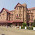# Xth CBSE Chemistry | NCERT Science Textbook - Chapter 1, Chemical Reactions and Equations | Notes and Sample Questions-Answers

Class 10, NCERT (CBSE) - Science (Chemistry)
Chapter 1, Chemical Reactions and Equations
Notes and Sample Questions
What is a ‘Reaction’?
What is a ‘Chemical Reaction’?
What is a ‘Chemical Equation’?
Types of Chemical Reaction
What are ‘Combination Reactions’?
What are ‘Decomposition Reactions’?
What are ‘Displacement Reactions’?
What are ‘Double Displacement Reactions’?
Q.1: Which of the following is a Combination and which is a Displacement Reaction?
(a) Cl2 + 2KI à 2KCl + I2
(b) 2K + Cl2 à 2KCl
Q.2: What is a catalytic decomposition?
Q.3: What is the term used for the symbolic representation of a reaction.
Q.4: Write two uses of decomposition reactions.
Q.5: Explain the terms ‘oxidizing agent’ and reducing agent’.
Further Study on Chapter 1, Chemical Reactions and Equations
• Chapter 1, Chemical Reactions and Equations | Class 10 CBSE Science (Chemistry) | NCERT Solution for In-Text Questions [Read]
• Class 10 NCERT (CBSE) Science Chemistry - Multiple choice questions (MCQ) | Chapter 1,  Chemical Reactions and Equations [Read]
• Class 10, CBSE (NCERT) Science | Chapter-1, Chemical Reactions and Equations | NCERT Solution - Science Textbook Exercise  [Read]

##### 3 comments:
Write comments
1.all those given are benefitial but if those not given are also provided means it will be more useful...............

2.can some one also post valencies of all elements.that would be useful

3.H-1
He-0
Li-1
Be-2
B-3
C-4
N-2
O-2
F-1
Ne-0
Na-1
Mg-2
Al-3
Si-4
P-3
S-2
Cl-1
Ar-0
K-1
Ca-2